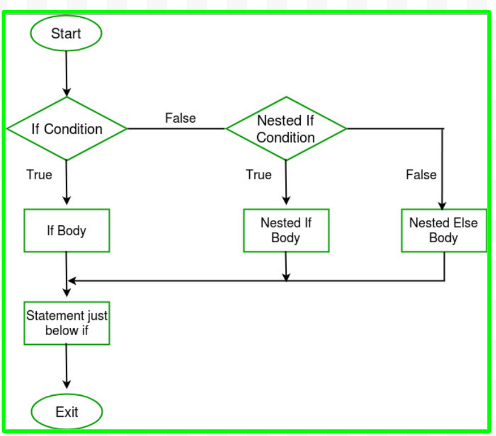GFG App
Open AppBrowser
Continue

If is a type of condition checking in which a condition turns out to be true a block of statements is executed.

Syntax:

```// if base_condition is true
// every inside the { } block will be executed
if (base_condition)
{
statement 1...............
statement 2 ..............
}```

Example

## C++

 `// C++ Program demonstrate` `// use if-else condition` `#include ` `using` `namespace` `std;`   `int` `main()` `{` `    ``int` `a = 6, b = 5;` `    ``if` `(a > b) {` `        ``cout << ``"True"` `<< endl;` `    ``}` `}`

Output

`True`

### What is Nested If?

When a number of if blocks are present one after another with the same scope (the same scope means under one { } block), then that condition is termed as a Nested if condition. If the first condition is True, we go into the next if condition and the subsequent condition is checked until we get a false condition, and the checking stops.

Syntax:

```// if base_condition is true control goes to base_condition1
if ( base_condition)
{
// if base_condition is true control goes to base_condition2
if(base_condition1)
{
if(base_condition2)
..........................
..........................
}
}```Example 1:

## C++

 `// C++ Program to` `// Nested-if conditions` `#include ` `using` `namespace` `std;`   `int` `main()` `{`   `    ``int` `a = 20, b = 10, c = 2;`   `    ``// if this condition satisfies then` `    ``// control goes to next if condition` `    ``if` `(a > b) {` `        ``// if this condition also turns out to be` `        ``// true then the statements under` `        ``// this block will get executed` `        ``if` `(a > c) {` `            ``cout << ``" a is the largest "` `<< endl;` `        ``}` `    ``}` `    ``return` `0;` `}`

Output

` a is the largest `

Example 2:

## C++

 `// C++ Program to` `// Nested-if conditions` `#include ` `using` `namespace` `std;`   `int` `main()` `{`   `    ``int` `a = 20, b = 10, c = 2;`   `    ``if` `(a == 20) {` `        ``if` `(b == 10) {` `            ``if` `(c == 2) {` `                ``cout << ``"Sandeep Sir is Great!!"` `<< endl;` `            ``}` `        ``}` `    ``}` `    ``return` `0;` `}`

Output

`Sandeep Sir is Great!!`

Example 3:

## C++

 `// C++ Program to` `// Nested-if conditions` `#include ` `using` `namespace` `std;`   `int` `main()` `{`   `    ``int` `a = 20, b = 10, c = 1;` `    ``// this condition is true` `    ``if` `(a == 20) {` `        ``// this condition is also true` `        ``if` `(b == 10) {` `            ``// but this condition is false hence` `            ``// we get out of the nested block` `            ``if` `(c == 2) {` `                ``cout << ``"Sandeep Sir is Great!!"` `<< endl;` `            ``}` `        ``}` `    ``}` `    ``cout << ``"gfg\n"``;`   `    ``return` `0;` `}`

Output

`gfg`

Example 4:

## C++

 `// C++ Program to demonstrate` `// Nested-if condition` `#include ` `using` `namespace` `std;`   `int` `main()` `{`   `    ``int` `a = 220, b = 10, c = 1;` `    ``// this condition is itself false we don't` `    ``// get inside the nesting if block` `    ``if` `(a == 20) {` `        ``if` `(b == 10) {` `            ``if` `(c == 2) {` `                ``cout << ``"Sandeep Sir is Great!!"` `<< endl;` `            ``}` `        ``}` `    ``}` `    ``cout << ``" No nested if condition is executed \n "``;` `    ``return` `0;` `}`

Output

``` No nested if condition is executed
```

Time complexity: O(1).

Auxiliary space: O(1).

My Personal Notes arrow_drop_up